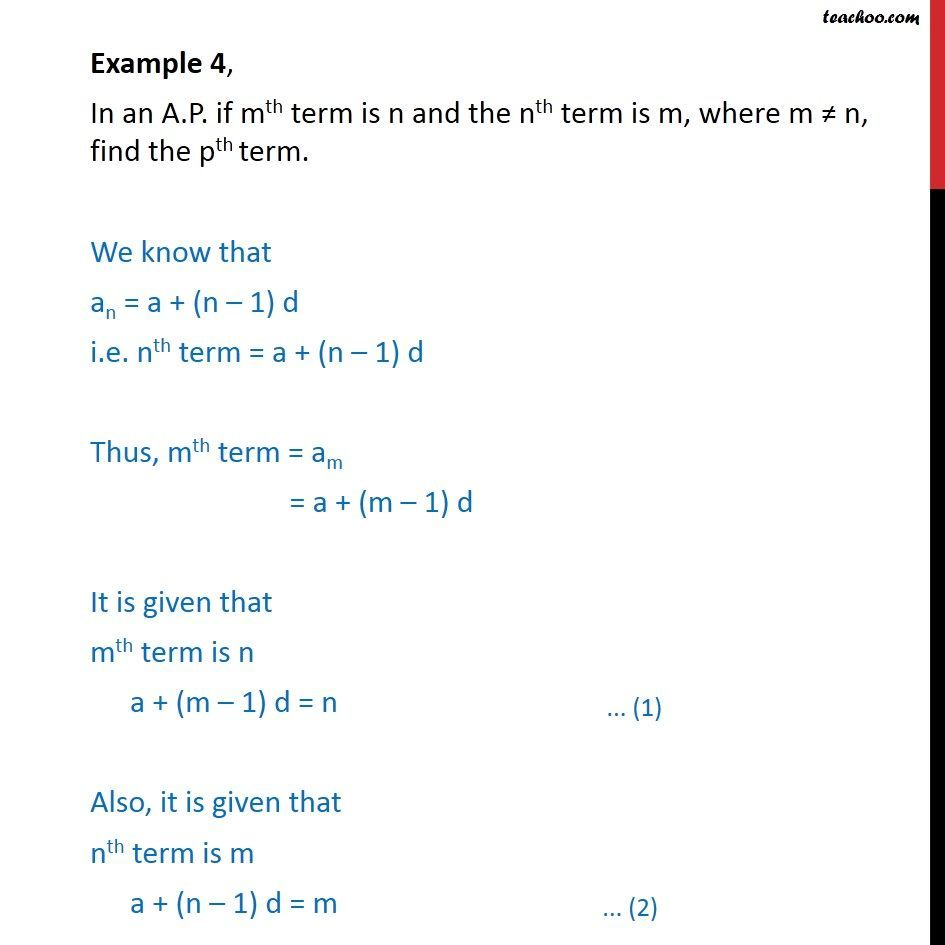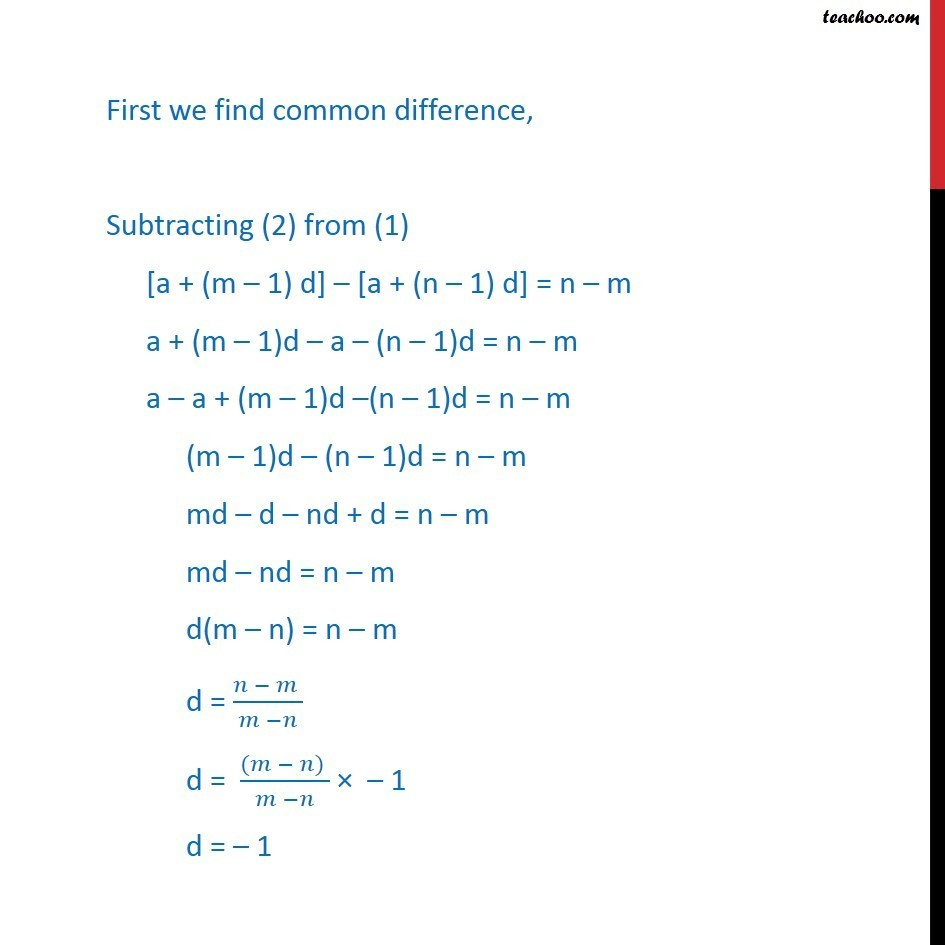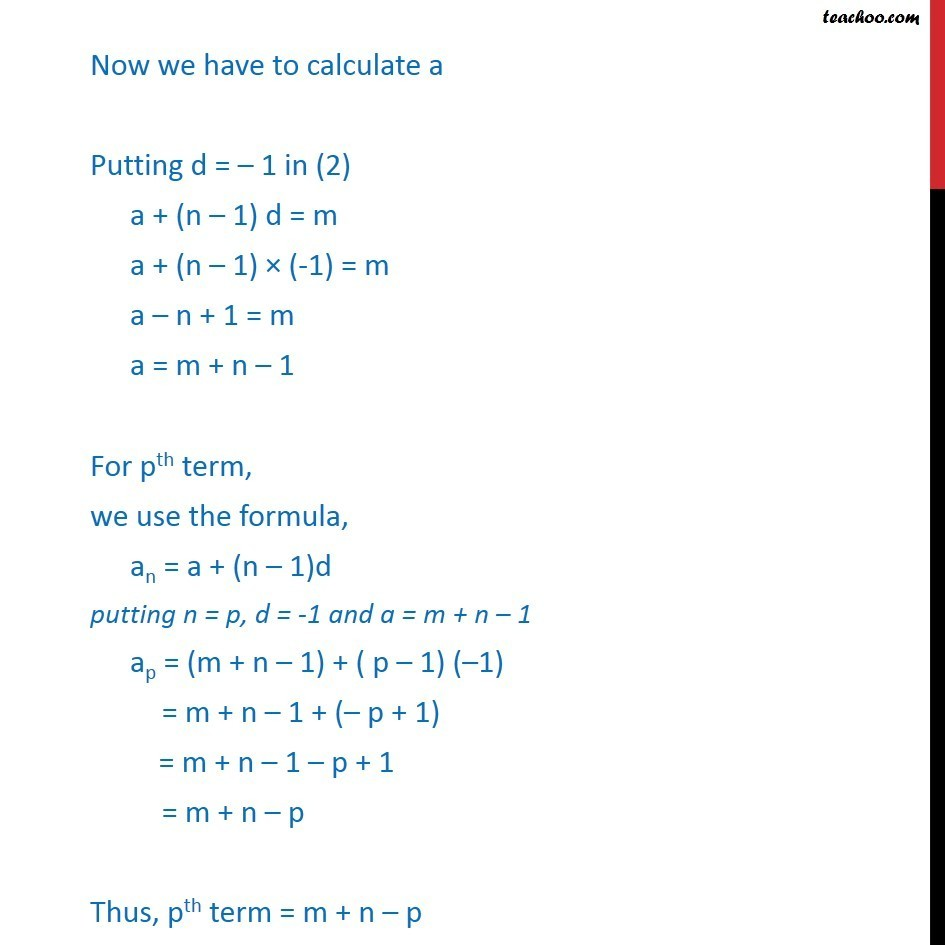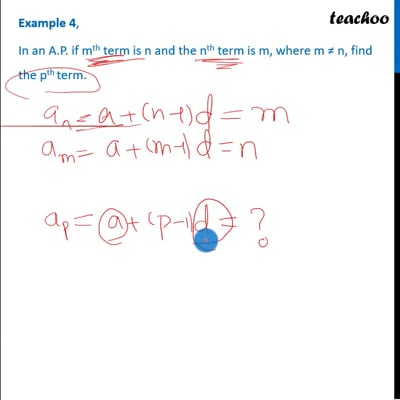Examples

Chapter 9 Class 11 Sequences and Series (Term 1)
Serial order wiseThis video is only available for Teachoo black users

### Transcript

Example 4, In an A.P. if mth term is n and the nth term is m, where m n, find the pth term. We know that an = a + (n 1) d i.e. nth term = a + (n 1) d Thus, mth term = am = a + (m 1) d It is given that mth term is n a + (m 1) d = n Also, it is given that nth term is m a + (n 1) d = m First we find common difference, Subtracting (2) from (1) [a + (m 1) d] [a + (n 1) d] = n m a + (m 1)d a (n 1)d = n m a a + (m 1)d (n 1)d = n m (m 1)d (n 1)d = n m md d nd + d = n m md nd = n m d(m n) = n m d = ( )/( ) d = (( ) )/( ) 1 d = 1 Now we have to calculate a Putting d = 1 in (2) a + (n 1) d = m a + (n 1) (-1) = m a n + 1 = m a = m + n 1 For pth term, we use the formula, an = a + (n 1)d putting n = p, d = -1 and a = m + n 1 ap = (m + n 1) + ( p 1) ( 1) = m + n 1 + ( p + 1) = m + n 1 p + 1 = m + n p Thus, pth term = m + n p